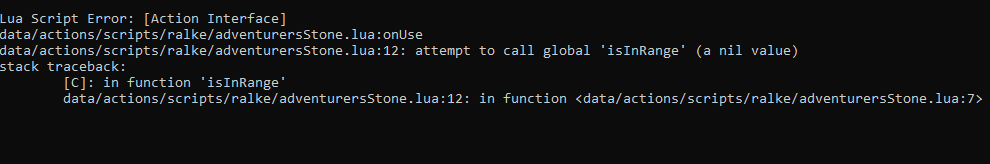# TFS 1.X+Attempt to call global 'isInRange'

#### ralke

##### (҂ ͠❛ ෴ ͡❛)ᕤ
Hi, i'm using TFS 1.4 downgraded by Nekiro. Got this script for the adventurer stone, but it has an error because is calling a nil value.Here is the script
Code:
``````local config = {
{fromPos = Position(1274, 967, 7), toPos = Position(1280, 973, 7), townId = 2},
{fromPos = Position(1008, 968, 7), toPos = Position(1026, 984, 7), townId = 1},
{fromPos = Position(1162, 1239, 6), toPos = Position(1169, 1244, 6), townId = 3}
}

function onUse(player, item, fromPosition, target, toPosition, isHotkey)

local playerPos, isInTemple, temple, townId = player:getPosition(), false
for i = 1, #config do
temple = config[i]
if isInRange(playerPos, temple.fromPos, temple.toPos) then
if Tile(playerPos):hasFlag(TILESTATE_PROTECTIONZONE) then
isInTemple, townId = true, temple.townId
break
end
end
end

if not isInTemple then
player:sendTextMessage(MESSAGE_EVENT_ADVANCE, 'Try to move more to the center of a temple to use the spiritual energy for a teleport.')
return true
end

player:setStorageValue(23352, townId)
playerPos:sendMagicEffect(CONST_ME_TELEPORT)

local destination = Position(238, 418, 7)
player:teleportTo(destination)
destination:sendMagicEffect(CONST_ME_TELEPORT)
return true
end``````

The functions isInRange is registered in core/position.lua
Code:
``````function Position:isInRange(from, to)
-- No matter what corner from and to is, we want to make
-- life easier by calculating north-west and south-east
local zone = {
nW = {
x = (from.x < to.x and from.x or to.x),
y = (from.y < to.y and from.y or to.y),
z = (from.z < to.z and from.z or to.z)
},
sE = {
x = (to.x > from.x and to.x or from.x),
y = (to.y > from.y and to.y or from.y),
z = (to.z > from.z and to.z or from.z)
}
}

if  self.x >= zone.nW.x and self.x <= zone.sE.x
and self.y >= zone.nW.y and self.y <= zone.sE.y
and self.z >= zone.nW.z and self.z <= zone.sE.z then
return true
end
return false
end``````

What I did wrong?

Solution
Hi, i'm using TFS 1.4 downgraded by Nekiro. Got this script for the adventurer stone, but it has an error because is calling a nil value.
View attachment 58749

Here is the script
Code:
``````local config = {
{fromPos = Position(1274, 967, 7), toPos = Position(1280, 973, 7), townId = 2},
{fromPos = Position(1008, 968, 7), toPos = Position(1026, 984, 7), townId = 1},
{fromPos = Position(1162, 1239, 6), toPos = Position(1169, 1244, 6), townId = 3}
}

function onUse(player, item, fromPosition, target, toPosition, isHotkey)

local playerPos, isInTemple, temple, townId = player:getPosition(), false
for i = 1, #config do
temple = config[i]
if isInRange(playerPos, temple.fromPos, temple.toPos) then
if...``````

#### Xikini

##### I whore myself out for likes
Support Team
Hi, i'm using TFS 1.4 downgraded by Nekiro. Got this script for the adventurer stone, but it has an error because is calling a nil value.
View attachment 58749

Here is the script
Code:
``````local config = {
{fromPos = Position(1274, 967, 7), toPos = Position(1280, 973, 7), townId = 2},
{fromPos = Position(1008, 968, 7), toPos = Position(1026, 984, 7), townId = 1},
{fromPos = Position(1162, 1239, 6), toPos = Position(1169, 1244, 6), townId = 3}
}

function onUse(player, item, fromPosition, target, toPosition, isHotkey)

local playerPos, isInTemple, temple, townId = player:getPosition(), false
for i = 1, #config do
temple = config[i]
if isInRange(playerPos, temple.fromPos, temple.toPos) then
if Tile(playerPos):hasFlag(TILESTATE_PROTECTIONZONE) then
isInTemple, townId = true, temple.townId
break
end
end
end

if not isInTemple then
player:sendTextMessage(MESSAGE_EVENT_ADVANCE, 'Try to move more to the center of a temple to use the spiritual energy for a teleport.')
return true
end

player:setStorageValue(23352, townId)
playerPos:sendMagicEffect(CONST_ME_TELEPORT)

local destination = Position(238, 418, 7)
player:teleportTo(destination)
destination:sendMagicEffect(CONST_ME_TELEPORT)
return true
end``````

The functions isInRange is registered in core/position.lua
Code:
``````function Position:isInRange(from, to)
-- No matter what corner from and to is, we want to make
-- life easier by calculating north-west and south-east
local zone = {
nW = {
x = (from.x < to.x and from.x or to.x),
y = (from.y < to.y and from.y or to.y),
z = (from.z < to.z and from.z or to.z)
},
sE = {
x = (to.x > from.x and to.x or from.x),
y = (to.y > from.y and to.y or from.y),
z = (to.z > from.z and to.z or from.z)
}
}

if  self.x >= zone.nW.x and self.x <= zone.sE.x
and self.y >= zone.nW.y and self.y <= zone.sE.y
and self.z >= zone.nW.z and self.z <= zone.sE.z then
return true
end
return false
end``````

What I did wrong?
Lua:
``if playerPos:isInRange(temple.fromPos, temple.toPos) then``

•ralke

#### ralke

##### (҂ ͠❛ ෴ ͡❛)ᕤ
Lua:
``if playerPos:isInRange(temple.fromPos, temple.toPos) then``
Thanks! Was wondering where to place player. Solved

•Xikini

Replies
8
Views
510
Replies
1
Views
221
Replies
15
Views
183
Replies
3
Views
256